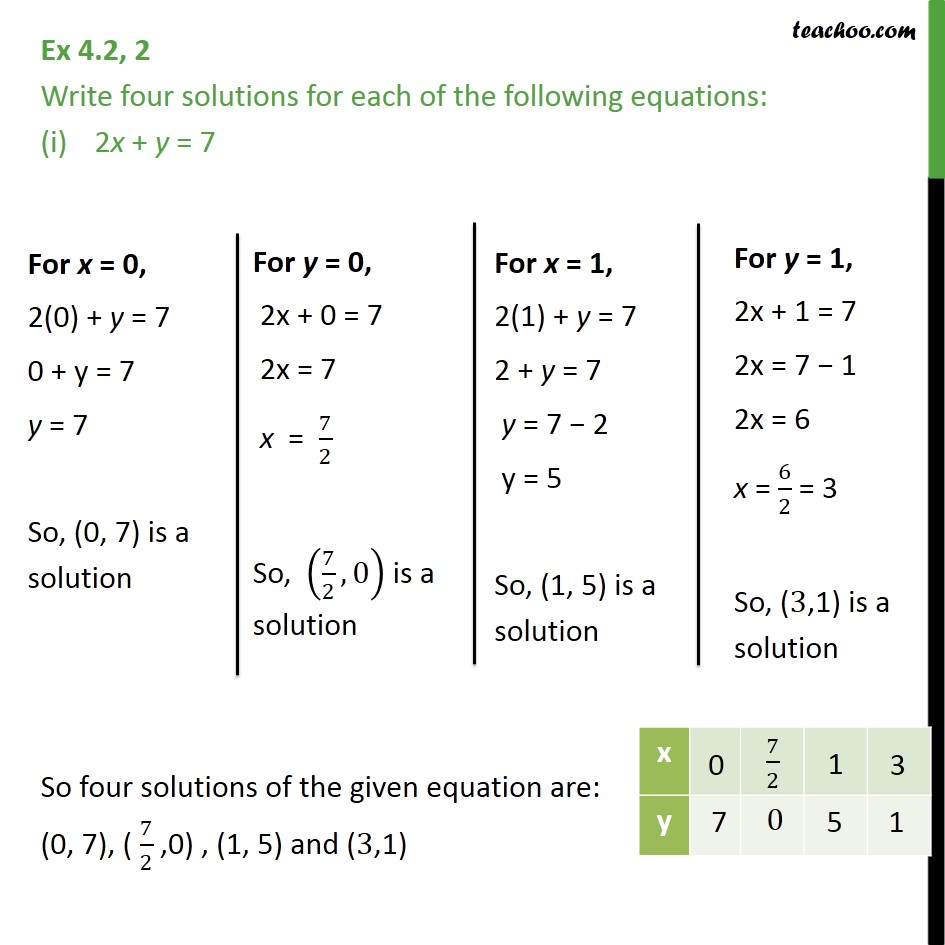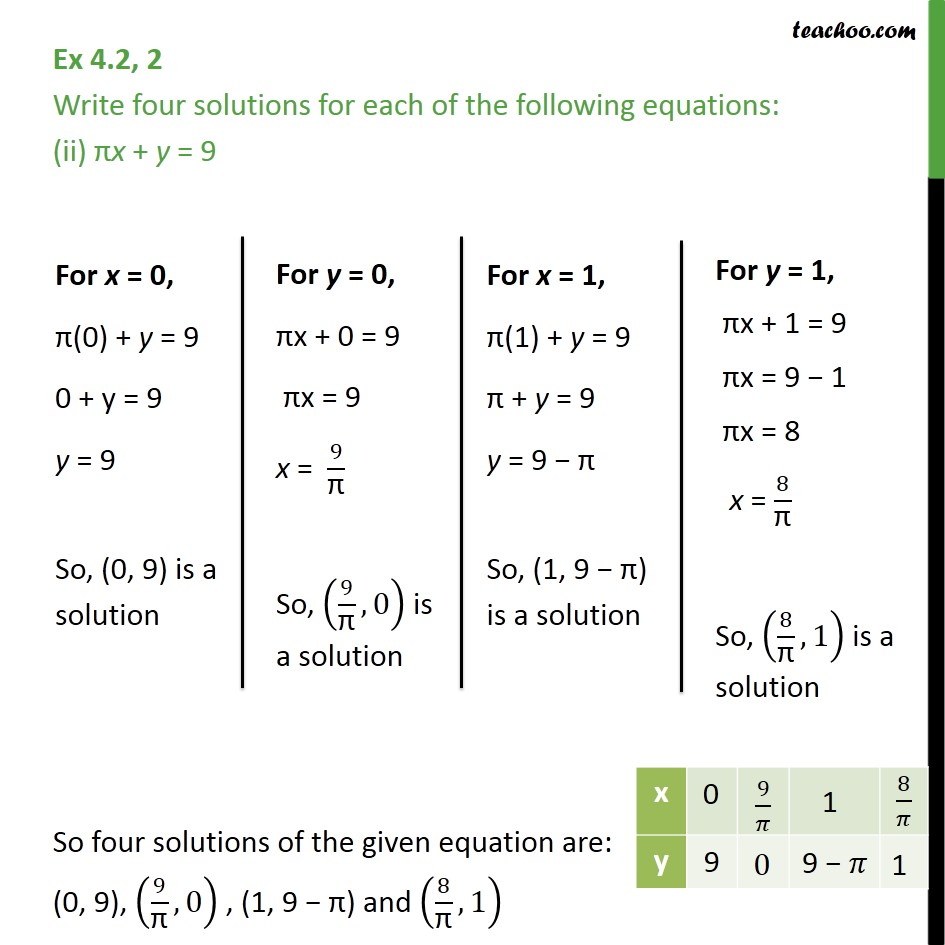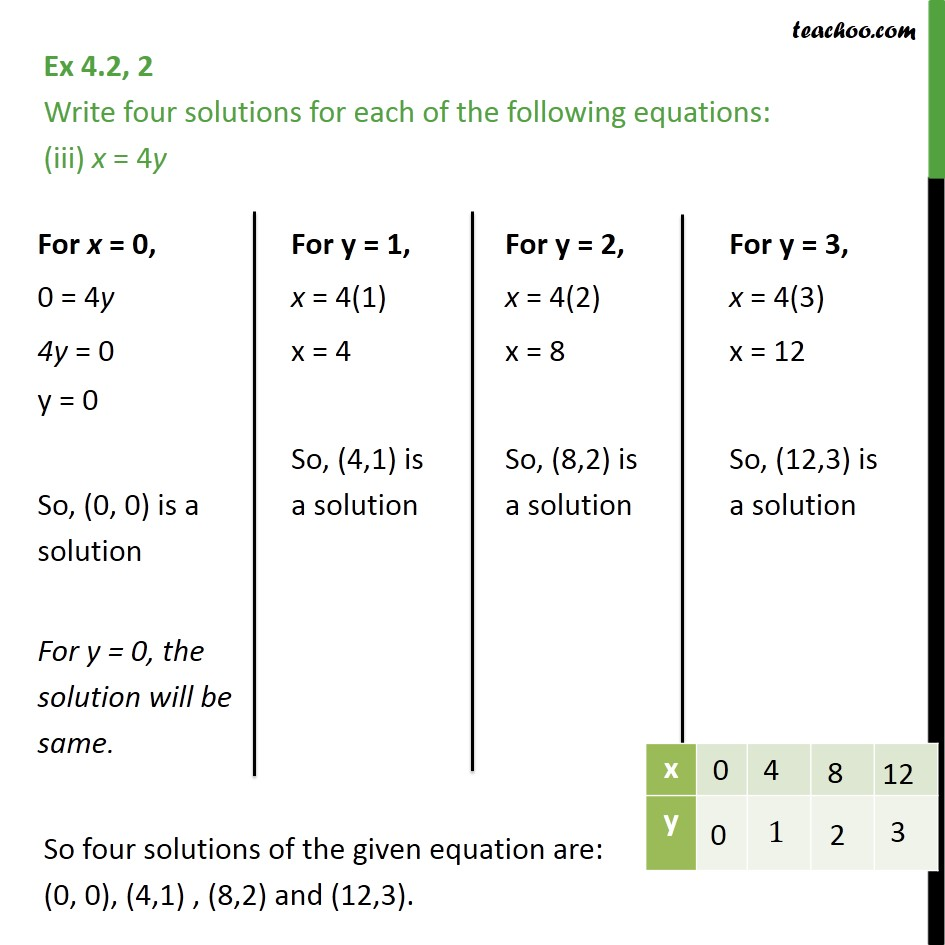Learn all Concepts of Linear Equations Class 9 (with VIDEOS). Check - Linear Equations in 2 Variables Class 91. Chapter 4 Class 9 Linear Equations in Two Variables
2. Serial order wise
3. Ex 4.2

Transcript

Ex 4.2, 2 Write four solutions for each of the following equations: 2x + y = 7 So four solutions of the given equation are: (0, 7), ( 7/2 ,0) , (1, 5) and (3,1) Ex 4.2, 2 Write four solutions for each of the following equations: (ii) x + y = 9 So four solutions of the given equation are: (0, 9), (9/" " ,0) , (1, 9 ) and (8/" " ,1) Ex 4.2, 2 Write four solutions for each of the following equations: (iii) x = 4y So four solutions of the given equation are: (0, 0), (4,1) , (8,2) and (12,3).

Ex 4.2